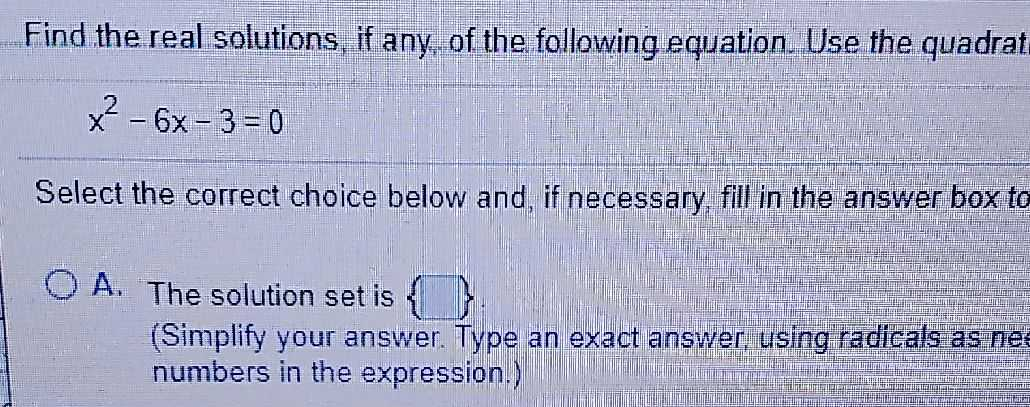### Still have math questions?

Algebra
QuestionFind the real solutions, if any. of the following equation. Use the quadrat

$$x ^ { 2 } - 6 x - 3 = 0$$

Select the correct choice below and, if necessary, fill in the answer box to A. The solution set is \{ \$$}$$ (Simplify your answer. Type an exact answer, using radicals as ner numbers in the expression.)

$$x = 3 + 2 \sqrt { 3 } , 3 - 2 \sqrt { 3 }$$
$$x = 6.46410161 \ldots , - 0.46410161 \ldots$$